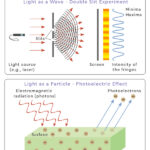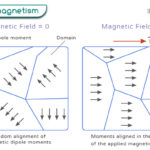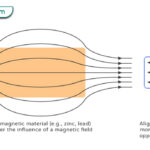Home / Physics / Ideal Gas

# Ideal Gas

An ideal gas is a hypothetical gas that is in close approximation to a real gas and obeys a specific set of laws. An ideal gas is suitable for modeling real gas and predicting the latter’s behavior.

## Properties of Ideal Gas

Since ideal gas is hypothetical, we make a few assumptions to consider a gas ideal.

1. The molecules do not interact. When they collide, the molecules do not attract or repel. The collisions are perfectly elastic, including the ones with the walls of the container.

2. The molecules do not occupy any volume and can be considered point particles far apart. However, the gas takes up the volume of the container.

3. The molecules exhibit continuous, rapid, and random motion. Since there is no interaction, the molecules can move independently, obeying Newton’s laws of motion.

4. The molecules possess kinetic energy but no potential energy. The average kinetic energy is proportional to the temperature of the gas.

5. The internal energy of an ideal gas is proportional to its temperature and independent of its volume.

## Equation Governing Ideal Gas Behavior

The thermodynamic state of an ideal gas can be described by a set of parameters known as state functions. These are pressure P, volume V, and temperature T. These quantities are related through a law known as the ideal gas law.

PV = nRT

Where

n: Number of moles of the gas

R: Universal gas constant (= 8.314 J mol-1 K-1)

Article was last reviewed on Saturday, February 11, 2023

### Related articlesWave-Particle DualityPhotoelectric EffectFerromagnetismDiamagnetism